Send us a message!+44 1(0)208 313 0992# Market Data Analysis and Charts using Python, Juypter Notebook and Pandas Data Frame### Pandas DataFrame and Jupyter Notebook

Pandas is an open-source Python library that lets you manipulate data quickly including storing it in various forms. Jupyter Notebook is an open-source web application that allows you to create documents and program various languages. Today we will look at how to get, change and store Forex data from a JSON API and in the process learn about Pandas and Jupyter Notebook.

Open your favourate browser and navigate to https://jupyter.org/try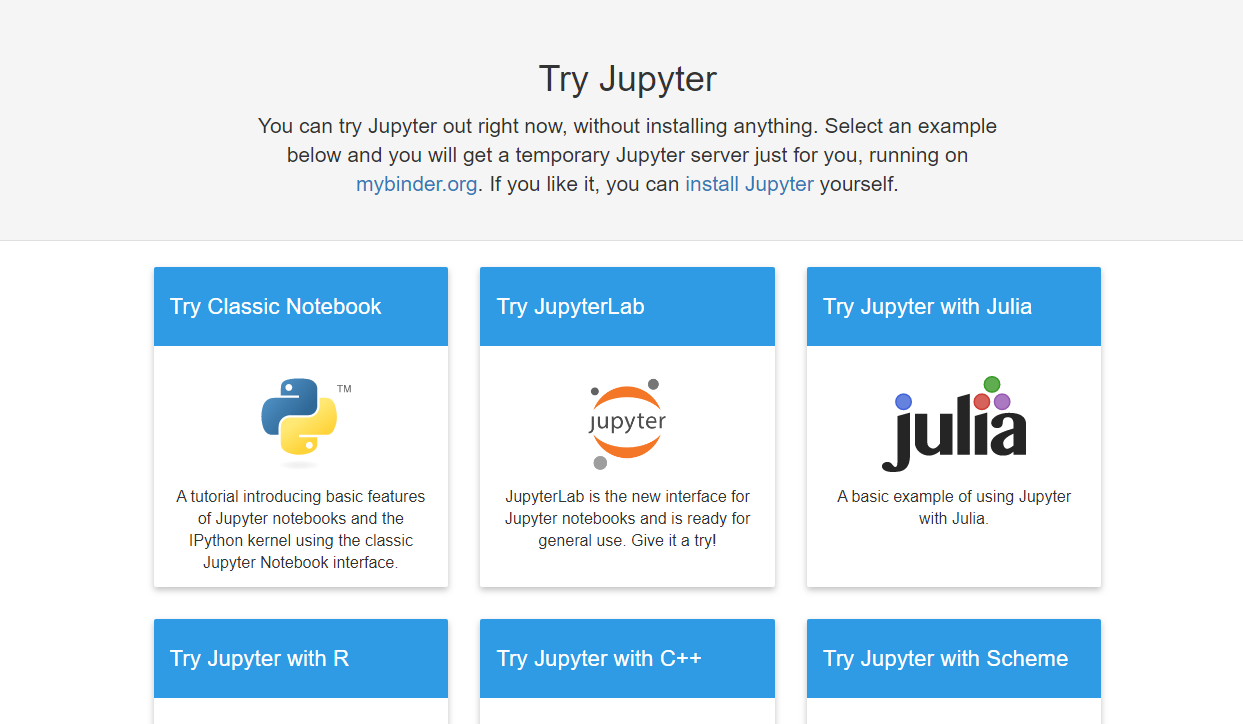Wait a few seconds at this will launch a Jupyter Notebook for you in your browser

Once you have started the notebook lets get an API key from https://tm-marketdata.com/signup the account is free for 30 days. This tutorial will work with other data services but the easiest path would be using our one. Once you have registered an account you will find you API Key under the myAccount page.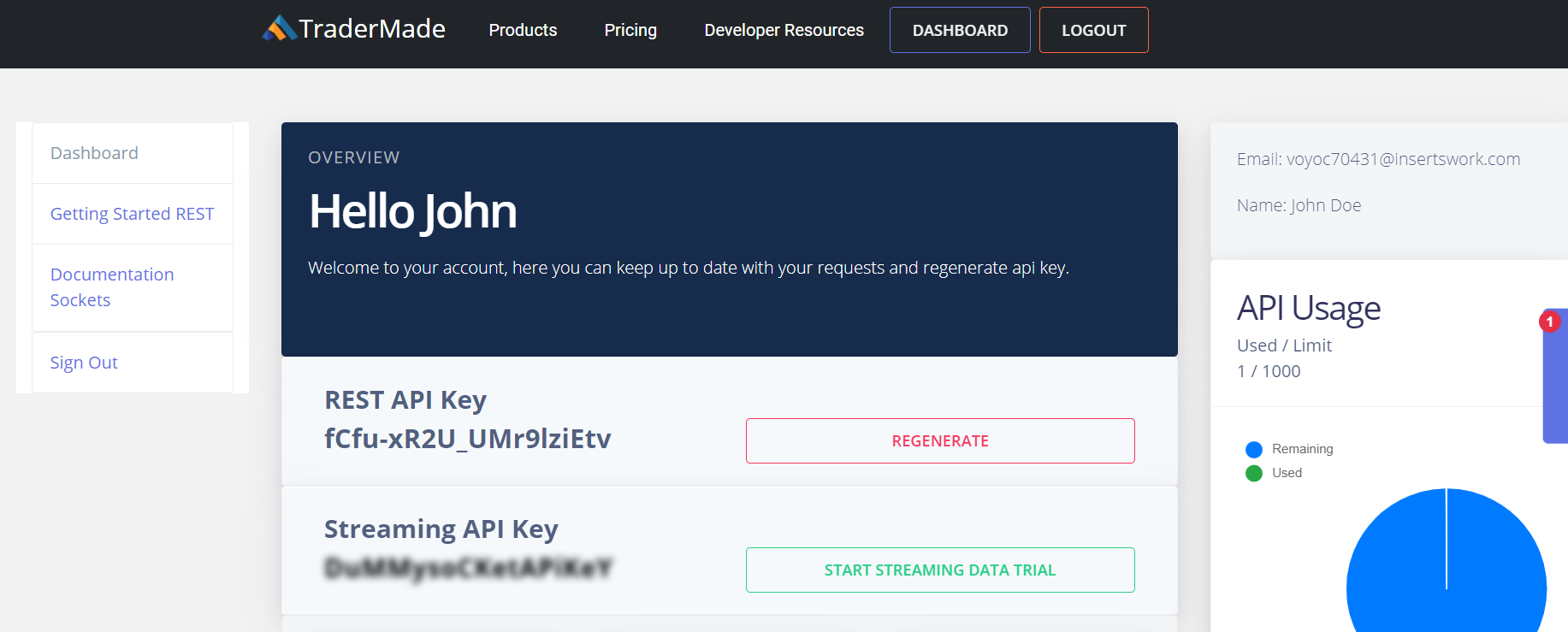Now we have the API Key we can get started

Click on the scissor sign (third from the left under Edit) three times this just clears out the welcome content so we can start coding.

#### Now lets write some code

In the cell write the following command and click run:

```                    ```
import pandas as pd
``````

This command imports the Pandas Data Frame for use in our notebook you can read more about Pandas here https://pandas.pydata.org

In the next cell type or copy the following variables, here we are setting up into settings we are going to pass in the data request. Don't forget to substitute you api_key.

```                    ```
currency = "EURUSD"
api_key = “paste your api key here”
start_date = "2019-10-01"
end_date= "2019-10-30"
format = "records"
fields = "ohlc"
```
```

Copy the following code, The first line of cope makes and request to the data server and formats the returned JSON data as a pandas Data Frame, the second line of code set the index for the data to the date field so it is displayed in date order, the third line of code "df" outputs the data frame in a nice format.

```                    ```
df = df.set_index("date")
df
```
```

Once you can copied the code and substituted you api_key you can run the program and you should get the following. The program has requested data from the data server written it into Pandas Data Frame format and output the data in a table format.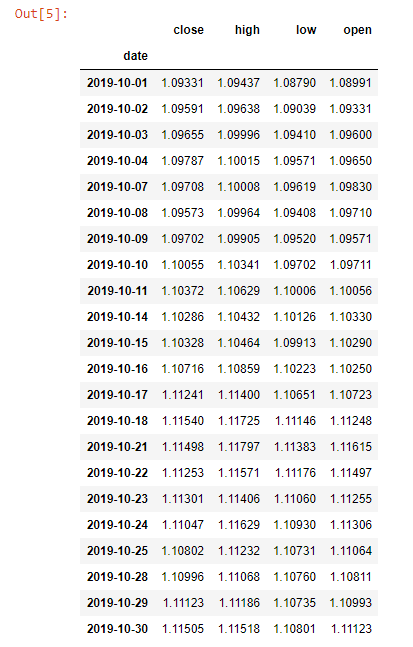As you can see we have got data for the period we specified in a data frame which is similar to a table but the cool thing about pandas is its ability to manipulate data and store data in various forms.

Before we dig deeper let’s just look a some basic command

df.head() - This will give us the first 5 lines of data

df.tail() - This will give us the last 5 lines of data

### Lets plot our data as a line chart

We can use the command df.plot(kind='line') and you will get the following chart, yes it really is that simple.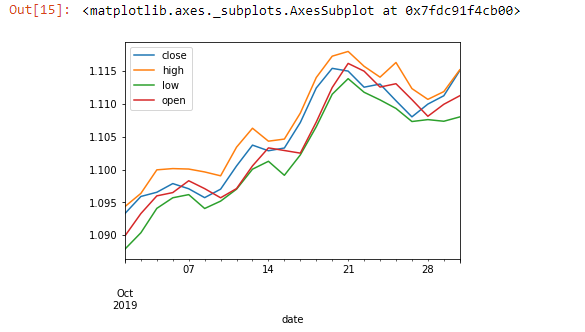The above command helps us visualize the data set.

Now let’s do something with our data set which will require a little effort in Excel. Let’s calculate the 5-day moving average:

```                    ```
df['5_day_MA'] =  df.close.rolling(5).mean()
df['5_day_volatility'] = df.close.rolling(5).std()
df = df.dropna()
```
```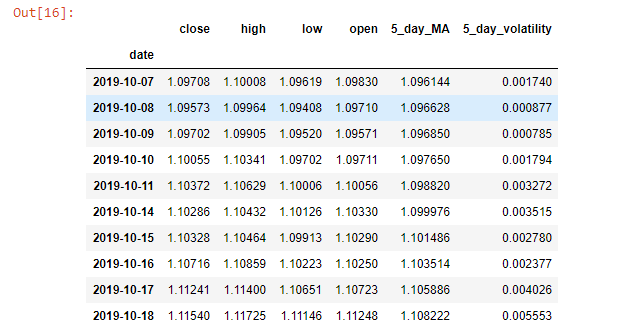As you can see dropna() function drops value that is N/A because we need at least 5 close days to get first moving average our data set is now from 07/10/2019

Plotting function can now let us see if the current data we pulled shows if the volatility is going up or down

```                    ```
df["5_day_volatility"].plot(title="EURUSD Volatility")
```
```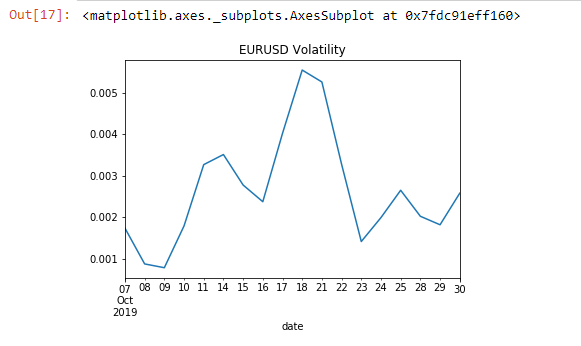So far we have looked at how a single data set works now let's use the API to compare two currencies

```                    ```
currency = "EURUSD,GBPUSD"
fields = "close"
"&end_date="+end_date+"&format="+format+"&fields="+fields)
df = df.set_index("date")
```
```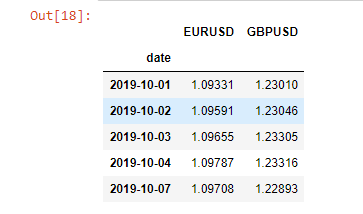We will first calculate pct_change for the currencies before seeing if there is any correlation between the two and then plot it.

```                    ```
df = df.pct_change(periods=1)
df = df.dropna()
df.corr()
```
```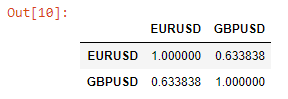Above function lets us see the daily correlation between EURUSD and GBPUSD over the whole data set. Next up we will see how this relationship is changing over time.

```                    ```
df["EURUSD_GBPUSD"] = df["EURUSD"].rolling(window=5).corr(other= df["GBPUSD"])
df = df.dropna()
df["EURUSD_GBPUSD"].plot(title="5 day correlation between EURUSD and GBPUSD")
```
```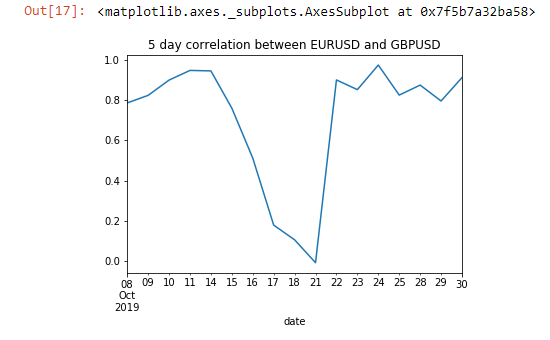Now we can see that there was a change in this correlation between 15th to the 21st of October.

If we have to save data in a CSV or JSON format all can also do that by

```                    ```
df.to_json(‘mydata.json’)
df.to_csv(‘mydata.csv’)
```
```

Now if you click File > open > myfile.json you can see the data is there. Also if you want to download just click the file and click download.

If you have any queries or question we are more than happy to answer queries via Chart or support@tradermade.com